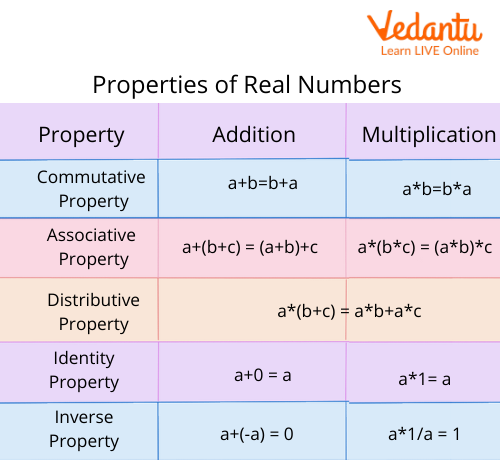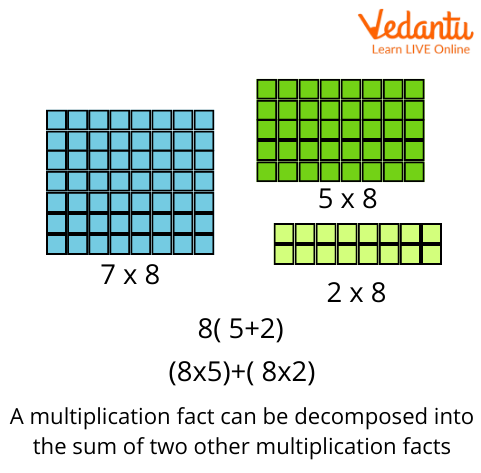Courses
Courses for Kids
Free study material
Free LIVE classes
More

# Understanding the Different Properties of MultiplicationLIVE
Join Vedantu’s FREE Mastercalss

## Properties of Multiplication for Kids Mathematics

Multiplication is an important aspect of Mathematics and involves different properties for solving differential equations. There are about six different properties of whole numbers which help in solving differential equations according to their rules. The six different properties of multiplication include the following:Properties of Real Numbers

The properties of multiplication have to go along with the properties of whole numbers, given above, to produce the appropriate answers and results. These properties provide accurate results when one has to solve for the long and bigger numbers. Let us dive into the properties of multiplication in detail in the next section.

## Closure Property for Multiplication

In easy words, the closure property states that if two whole numbers say, a and b are multiplied together, a x b then the result of the multiplication will also be a whole number.

It's a rule that when whole numbers are multiplied then the result will also be produced as a whole number. Hence, to verify this property of multiplication, let's look at the examples.

Example: 8x9= 72

Here, both 8 and 9 are whole numbers and the result, 72 is also considered to be a whole number.

Some other examples include:

• 2x5= 10

• 5x7= 35

• 7x11= 77

## Commutative Property for Multiplication

The commutative property states that the order of two multiplicative numbers does not change the product and the answers remain the same even if their orders are changed.

a x b = b x a

Example: 6 x 3= 18 & 3 x 6 = 18

Hence, we can prove here that the numbers multiplied in either way produce the same results irrespective of their places.

Some more of the examples include:

• 7 x 8 = 56 & 8 x 7 = 56

• 12 x 2 = 24 & 2 x 12 = 24

• 7 x 6 = 42 & 6 x 7 = 42

## Multiplication by Zero

The property of multiplication by zero states that if any number is multiplied by zero the result is always zero.

a x 0 = 0 or 0 x a = 0

Example: 22 x 0 = 0 or 0 x 22 = 0

Therefore, the result proves that any number multiplied with zero irrespective, the result is always supposed to be 0.

Some other examples include:

• 37 x 0 = 0 & 0 x 37 = 0

• 4 x 0 = 0 & 0 x 4 = 0

## Identity Property of Whole Numbers

This property states that if any whole number when multiplied by 1 produces the number as result itself.

Considering if 'a' is a whole number, which is multiplied by 1, we will get:

1 x a = a or a x 1 = a

Example:

• 1 x 7 = 7 ( 1+1+1+1+1+1+1 = 7)

• 2 x 1 = 2 ( 1+1 = 2)

• 1 x 342 = 342

The number 1 is considered as the multiplicative identity for the whole numbers since it does not change the value of numbers.

## Associative Property of Multiplication

The associative property involves the multiplication of three whole numbers. It states that if any three whole numbers are multiplied in any manner or order, the answer always remains the same.

Let, a, b and c be three whole numbers:

(a x b) x c = a x (b x c)

The associative property ensures that the product or the answer of the multiplication remains the same irrespective of the placement of the numbers. The associative property applies to three or more numbers during multiplication.

Example:

• (2 x 3) x 6 = 36

• (3 x 6) x 2 = 36

• (6 x 2) x 3 = 36

## Distributive Property of Multiplication

The distributive property states that when the addition of two numbers is multiplied by the third number, the answer is always equal to the sum of two products.

Let a, b, and c be three whole numbers:

a x (b + c) = a x b + a x c

Thus, we say that the multiplication of numbers distributes over the addition of their numbers.Distributive Property

Example:

• 2 x (7 + 3) = 20 and 2 x 7 + 2 x 3 = 20

• 3 x (1 + 4) = 15 and 3 x 1 + 3 x 4 = 15

## Summary

The different properties of multiplication help us to solve elaborate questions efficiently and with accuracy. These different properties are quick and easy to use and can be used in different methods. We hope you now have a clear understanding of what the different properties of multiplication are and can apply them to accurately while solving problems. You can visit our website to get hold of worksheets on the topic or access other interesting Maths topics.

Last updated date: 25th Sep 2023
Total views: 125.7k
Views today: 2.25k

## FAQs on Understanding the Different Properties of Multiplication

1. Integers involve which of the different operations?

An integer is colloquially defined as a number that can be written without a fractional component. Integers involve the basic operations of addition, subtraction, multiplication, and division. These basic operations are used throughout the work of mathematics and help solve various sets of questions and equations. These operations are also the main segments which come to use in any part of maths even if the question is simple or complicated. One can use these operations to solve large sets of questions efficiently and in no time.

2. What is the equation for the commutative property of multiplication?

The equation for the commutative property of multiplication is considered to be: a x b = b x a. According to the commutative property of multiplication, the answer of the multiplication remains the same even if the order of the numbers multiplying changes.

For example: 7 x 4 = 28 and 4 x 7 = 28

One can see how the places of 4 and 7 have been interchanged yet their result comes as 28 in both cases.